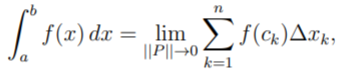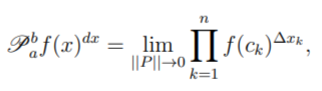# Product Integral

Generally speaking, product integrals have multiplication instead of summation. They were originally introduced for solving differential equations.

Product integrals are used in a wide variety of areas including fluid mechanics, probability theory (especially survival analysis and Markov processes) and mathematical analysis.

## Product Integral vs. Ordinary Integral

An ordinary integral (i.e. the “usual” integral that involves summations) gives the solution of the equation
y′(x) = f(x)
while the product integral can find solutions for equations of the form
y′(x) = A(x)y(x).
A can be a scalar function or a matrix function. If A is a matrix function then y is a vector function and the equation above represents a system of linear differential equations of the first order. (Slavík, 2007).

## Definition of a Product Integral

A product integral can be defined in several different ways, mostly using matrices (Dudley, 2006). One fairly straightforward way to define it is similar to how the product derivative is defined.

The familiar Riemann integral of f over some closed interval [a, b] is given by:Where:

• P = a partition of the interval [a, b]
• ||P|| = the maximum value of Δxk (the width of the largest subinterval in P)
• ck = any point in [xk-1, xk]

The product derivative is derived from the definition of the usual derivative: subtraction is changed to division and division is changed to taking roots. The Riemann product integral can be defined in a similar way. The definition for the Riemann integral, given above, is changed as follows:

• Addition is changed to multiplication,
• Multiplication is changed to exponentiation.

This gives the Riemann product integral of f over [a, b]:## References

Dudley, R. et al. (2006). Differentiability of Six Operators on Nonsmooth Functions and P-Variation. Springer Berlin Heidelberg.
Slavík, A. (2007). Product Integration, It’s History and Applications. NEXAS Center for Mathematical Modeling, Volume 1: History of Mathematics, Volume 29.

CITE THIS AS:
Stephanie Glen. "Product Integral" From StatisticsHowTo.com: Elementary Statistics for the rest of us! https://www.statisticshowto.com/product-integral/